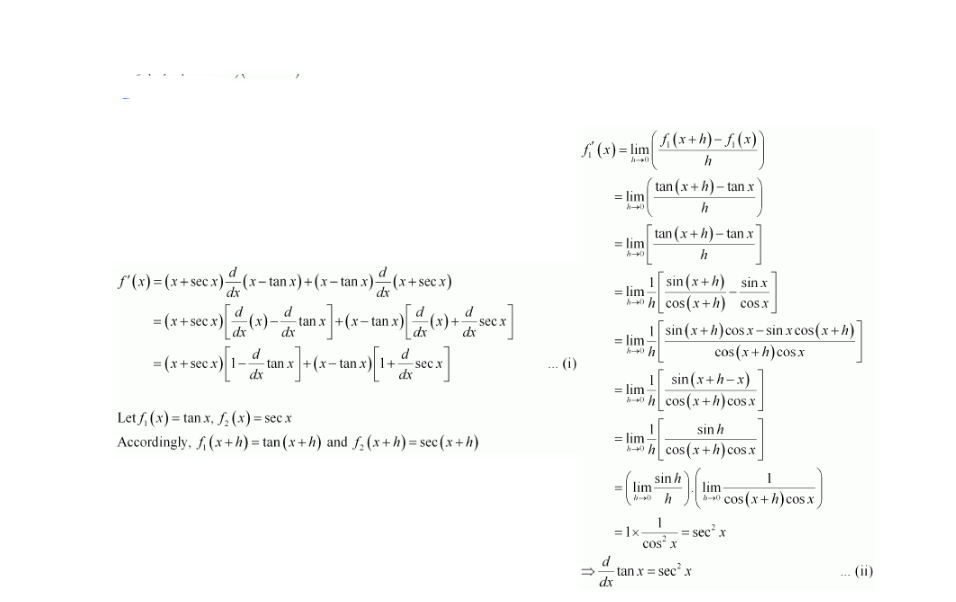# Find the derivative of the following functions

Question:

Find the derivative of the following functions (it is to be understood that abcdp, q, r and s are fixed non-zero constants and m and n are integers): (x + sec x) (x – tan x)

Solution:

Let $f(x)=(x+\sec x)(x-\tan x)$

By product rule,$f_{2}^{\prime}(x)=\lim _{h \rightarrow 0}\left(\frac{f_{2}(x+h)-f_{2}(x)}{h}\right)$

$=\lim _{h \rightarrow 0}\left(\frac{\sec (x+h)-\sec x}{h}\right)$

$=\lim _{h \rightarrow 0} \frac{1}{h}\left[\frac{1}{\cos (x+h)}-\frac{1}{\cos x}\right]$

$=\lim _{h \rightarrow 0} \frac{1}{h}\left[\frac{\cos x-\cos (x+h)}{\cos (x+h) \cos x}\right]$

$=\frac{1}{\cos x} \cdot \lim _{h \rightarrow 0} \frac{1}{h}\left[\frac{-2 \sin \left(\frac{x+x+h}{2}\right) \cdot \sin \left(\frac{x-x-h}{2}\right)}{\cos (x+h)}\right]$

$=\frac{1}{\cos x} \cdot \lim _{h \rightarrow 0} \frac{1}{h}\left[\frac{-2 \sin \left(\frac{2 x+h}{2}\right) \cdot \sin \left(\frac{-h}{2}\right)}{\cos (x+h)}\right]$

$=\frac{1}{\cos x} \cdot \lim _{h \rightarrow 0}\left[\frac{\sin \left(\frac{2 x+h}{2}\right)\left\{\frac{\sin \left(\frac{h}{2}\right)}{\frac{h}{2}}\right\}}{\cos (x+h)}\right]$

$=\sec x \frac{\left\{\lim _{h \rightarrow 0} \sin \left(\frac{2 x+h}{2}\right)\right\}\left\{\lim _{\frac{h}{2} \rightarrow 0} \frac{\sin \left(\frac{h}{2}\right)}{\frac{h}{2}}\right\}}{\lim _{h \rightarrow 0} \cos (x+h)}$

$=\sec x, \frac{\sin x .1}{\cos x}$

$\Rightarrow \frac{d}{d x} \sec x=\sec x \tan x$ ...(iii)

From (i), (ii), and (iii), we obtain

$f^{\prime}(x)=(x+\sec x)\left(1-\sec ^{2} x\right)+(x-\tan x)(1+\sec x \tan x)$